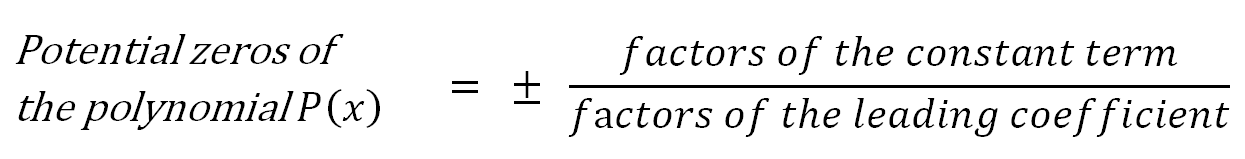# Rational zero theorem

### Rational zero theorem

What is rational zeros theorem? It is sometimes also called rational zero test or rational root test. We can use it to find zeros of the polynomial function. It is used to find out if a polynomial has rational zeros/roots. It also gives a complete list of possible rational roots of the polynomial. It also comes in handy when we need to factor a polynomial alongside with the use of polynomial long division or synthetic division.

#### Lessons

Rational Zero Theorem:• Introduction
Introduction to Rational Zero Theorem

• 1.
Consider the polynomial $P\left( x \right) = 9{x^3} + 6{x^2} - 29x - 10$
a)
Determine the potential zeros

b)
Factor $P\left( x \right)$ fully.

c)
Solve the equation: $9{x^3} + 6{x^2} - 29x - 10 = 0$

• 2.
Solve the equation: ${x^4} - 5{x^3} + 22x - 12 = 0$